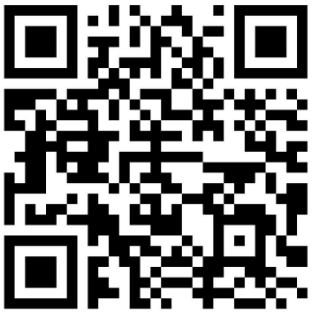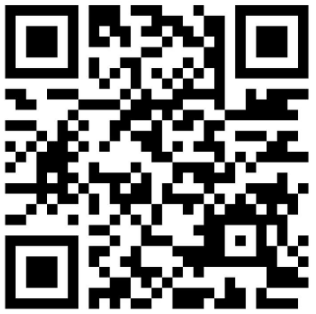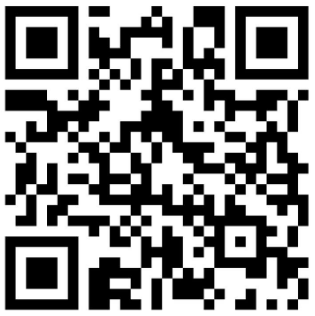## Bitcoin WIF

Wallet Import Format (WIF, also known as Wallet Export Format) is a way of BASE58 encoding a private ECDSA key so as to make it easier to copy. https://en.bitcoin.it/wiki/Wallet_import_format

### Find WIF From Private Key

```<?php

use BitWasp\Bitcoin\Bitcoin;
use BitWasp\Bitcoin\Network\NetworkFactory;
use BitWasp\Bitcoin\Key\Factory\PrivateKeyFactory;

if (\$_SERVER['REQUEST_METHOD'] == 'POST') {
try {
\$networkClass   = \$_POST['network'];
Bitcoin::setNetwork(NetworkFactory::\$networkClass());
\$network        = Bitcoin::getNetwork();
\$privKeyFactory = new PrivateKeyFactory();

if (\$_POST['compression'] == 'y') {
\$privateKey = \$privKeyFactory->fromHexCompressed(\$_POST['privkey']);
} else {
\$privateKey = \$privKeyFactory->fromHexUncompressed(\$_POST['privkey']);
}

?>
<div class="table-responsive">
<table border=0 class='table'>
<tr><td>Wif</td><td><?php echo \$privateKey->toWif()?></td></tr>
</table>
</div>
<?php
} catch (Exception \$e) {
\$errmsg .= "Problem found. " . \$e->getMessage();
}
}

if (\$errmsg) {
?>
<strong>Error!</strong> <?php echo \$errmsg?>
</div>
<?php
}
?>
<form action='' method='post'>
<div class="form-group">
<label for="network">Network:</label>
<select id="network" name="network" class="form-control" >
<?php
\$networks = get_class_methods(new NetworkFactory());
foreach(\$networks as \$network) {
echo "<option value='{\$network}'".(\$network == \$_POST['network'] ? " selected": "").">{\$network}</option>";
}
?>
</select>
</div>
<div class="form-group">
<label for="privkey">Private Key (Hex):</label>

<input class="form-control" type='text' name='privkey' id='privkey' value='<?php echo \$_POST['privkey']?>'>

</div>
<div class="form-group">
<label for="compression" >Compression:</label>
<select name="compression" class="form-control" id='compression'>
<?php
\$yesno = array("y"=>"Yes", "n"=>"No");
foreach(\$yesno as \$yesno_k=>\$yesno_v) {
echo "<option value='{\$yesno_k}'".(\$yesno_k == \$_POST['compression'] ? " selected": "").">{\$yesno_v}</option>";
}
?>
</select>
</div>
<input type='submit' class="btn btn-success btn-block"/>
</form>
<?php
include_once("html_iframe_footer.php");		```

### Find Private Key From WIF

```<?php

use BitWasp\Bitcoin\Bitcoin;
use BitWasp\Bitcoin\Network\NetworkFactory;
use BitWasp\Bitcoin\Key\Factory\PrivateKeyFactory;

if (\$_SERVER['REQUEST_METHOD'] == 'POST') {
try {
\$networkClass   = \$_POST['network'];
Bitcoin::setNetwork(NetworkFactory::\$networkClass());
\$network        = Bitcoin::getNetwork();
\$privKeyFactory = new PrivateKeyFactory();
\$privateKey = \$privKeyFactory->fromWIF(\$_POST['wif'], \$network);
?>
<div class="table-responsive">
<table border=0 class='table'>
<tr><td>Private Key Hex</td><td><?php echo \$privateKey->getHex()?></td></tr>
</table>
</div>
<?php
} catch (Exception \$e) {
\$errmsg .= "Problem found. " . \$e->getMessage();

}
}

if (\$errmsg) {
?>
<strong>Error!</strong> <?php echo \$errmsg?>
</div>
<?php
}
?>
<form action='' method='post'>
<div class="form-group">
<label for="network">Network:</label>
<select id="network" name="network" class="form-control" >
<?php
\$networks = get_class_methods(new NetworkFactory());
foreach(\$networks as \$network) {
echo "<option value='{\$network}'".(\$network == \$_POST['network'] ? " selected": "").">{\$network}</option>";
}
?>
</select>
</div>
<div class="form-group">
<label for="wif">Wif:</label>
<input class="form-control" type='text' name='wif' id='wif' value='<?php echo \$_POST['wif']?>'>
</div>
<input type='submit' class="btn btn-success btn-block"/>
</form>
<?php
include_once("html_iframe_footer.php");		```

##### Tutorials